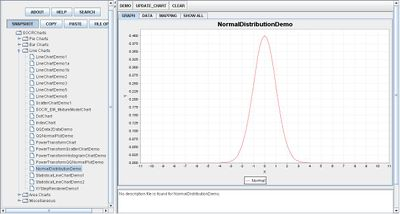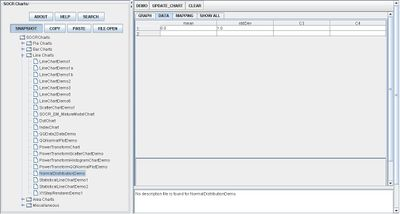# Difference between revisions of "SOCR EduMaterials Activities NormalDistribution"

## Summary

This activity describes the need, general methods and SOCR utilities for the normal distribution. The simulated data may then be easily copied and pasted in different SOCR Analyses or Graphing tools for further interrogation.

## Goals

The aims of this activity are to:

• strengthen one's knowledge of the normal distribution
• show how SOCR normal distribution may be used as an advantage
• compare the use of the normal distribution to other areas of study

## Background

Also known as the Gaussian Distribution, the Normal Distribution is often associated with the bell curve because the graph resembles the shape of a bell. The Normal Distribution is essential when modeling quantitative data in natural environments. Statistical data may be implemented in these graphs as the mean, variance, and standard deviation may be labeled.

For references, visit Wikipedia and WolFram.

## Description

Go to the SOCR Charts and select Line Charts from the items located on the left, then select the demonstration for Normal Distribution:The image above is a demonstration of a Normal Distribution. Notice the bell shaped curve that is centered at 0 on the horizontal axis.

## Examples & Exercises

• Exercise 1: Does it matter if the variables are random or chosen when using the normal distribution? Why or why not?
• Exercise 2: How can the normal distribution show the probability of one event from occurring just by the display?
• Exercise 3: What other type of chart may be associated with the normal distribution when displaying the outcome of a probability experiment?

## Data Type and Format

By clicking Data between the Graph and Mapping button, it allows users to input or vary the values of the data set. For the Normal Distribution, the mean and standard deviation are the only two important values in the table. Adjusting the mean will shift the graph and varying the standard deviation will change the range or horizontal length of the graph:## Applications

One of the most persuasive elements when proposing data and literature to others is a well-designed chart presentation.

Suppose a professor wants to grade his students based on the Normal Distribution Graph in which a small percentage of the class will receive A’s and F’s while the majority of the class will receive C’s.